Next: MINIMUM NETWORK INHIBITION ON Up: Cuts and Connectivity Previous: MAXIMUM DIRECTED CUT   Index

### MAXIMUM K-CUT

• INSTANCE: Graph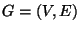, a weight function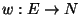, and an integer.

• SOLUTION: A partition of V into k disjoint sets.

• MEASURE: The sum of the weight of the edges between the disjoint sets, i.e.,• Good News: Approximable within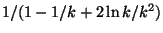and .

• Comment: The unweighted version is equivalent to MAXIMUM K-COLORABLE SUBGRAPH. Approximable within 1.21 for k=3, 1.18 for k=4, and 1.15 for k=5 . Not approximable within 1+1/(34k) . MAXIMUM K-SECTION, the problem with the additional constraint that the partition must cut the graph into sets of equal size, is approximable within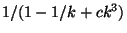for some constant c>0 .
The constrained variation in which the input is extended with a positive integer W, a vertexand a subset S of V, and the problem is to find the 2-cut of weight at least W with the largest number of vertices from S on the same side as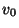, is not approximable withinfor some.